The data in Table 3 are the birth weights (in kg) of 50 infants suffering from severe idiopathic respiratory distress syndrome. There are two groups of infants: those who survived the condition (there were 23 of these) and those who, unfortunately, did not. The data have not been sor
Author(s): The Open University

## Activity 21

Without using your calculator solve the following calculations.

• (a) 3 + 5 × 2 = ?

• (b) 12 − 6 + 6 = ?

• (c) 6 + (5 +
Author(s): The Open University

When calculating an answer it is important that you give careful consideration to the order of operations used in the calculation. If you are using a mixture of operations remember that certain operations take priority in a calculation. Consider the following, apparently, simple sum.

1 + 2 × 3 = ?

Did you give 7 as your response, or 9?

The correct answer is 7 but can you explain why?

If you have a calculator handy, check that it
Author(s): The Open University

After studying this course, you should be able to:

• round a given whole number to the nearest 10, 100, 1000 and so on

• round a decimal number to a given number of decimal places or significant figures

• use rounded numbers to find rough estimates for calculations

• use a calculator for decimal calculations involving +, −, × and ÷, giving the answer to a specified accuracy (e.g. decimal places or significant figures) and checking the ans
Author(s): The Open University

In the last subsection we stated that, for any integer n ≥ 2, the set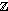n satisfies the same rules for addition modulo n as the real numbers satisfy for ordinary addition. When it comes to multiplicat
Author(s): The Open University

The Division Algorithm tells us that all the possible remainders on division by an integer n lie in the set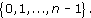We denote this set by [Image_Link]https://www.open.edu/
Author(s):
The Open University

In OpenLearn course Diagrams, graphs and charts you saw how a scale is used on plans of houses and other structures. The scale makes it possible to take a length on the plan and calculate the correspond
Author(s): The Open University

Two straight lines that do not intersect, no matter how far they are extended, are said to be parallel. Arrows are used to indicate parallel lines.Author(s): The Open University

## Question 1

Calculate all the angles at the centres of these objects.

Here is an improved solution which shows working.

## Example 13

Suppose you plan to redecorate your bathroom. The end wall has the following shape, with dimensions as shown on the diagram. The quality of the plasterwork is not good and you are considering tiling the wall.

Author(s): The Open University

## Example 14

Suppose you have decided to tile the wall using square tiles of side 10 cm. You are proposing to use the tiles across the full 5 metre width of the wall up to a height of 1.8 m.

Find the number of boxes of tiles that you will require to cover the wall if the tiles a
Author(s): The Open University

The modulus function provides us with a measure of distance that turns the set of complex numbers into a metric space in much the same way as does the modulus function defined on R. From the point of view of analysis the importance of this is that we can talk of the closeness of two complex numbers. We can then define the limit of a sequence of complex numbers in a way which is almost identical to the definition of the limit of a real sequence. Another analogue of real analysis arises
Author(s): The Open University

You will no doubt recall that in real analysis extensive use is made of the modulus function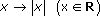. It gives us a way of measuring the “closeness” of two numbers, which we exploit in writing expressi
Author(s): The Open University

In this section we have seen a correspondence between complex numbers and points in the plane using Cartesian coordinates; the real part of the complex number is represented on the real axis (“horizontal”) and the imaginary part on the imaginary axis (“vertical”). We can also use polar coordinates (r,θ) in which case r, the modulus of a non-zero complex number z is positive and θ is an argument of z, defined only to within an additive intege
Author(s): The Open University

We have seen in Section 2.2 that the complex number system is obtained by defining arithmetic operations on the set R × R. We also know that elements of R × R can be represented as points in a plane. It seems reasonable to ask what insight can be obtained by representing complex numbers as
Author(s): The Open University## Study another free course

There are more than 800 courses on OpenLearn for you to
Author(s): The Open University

In Section 5 we show how functions may be used to sketch curves in the plane, even when these curves are not necessarily the graphs of functions.

Click the link below to open Section 5 (8 pages, 151KB).

Section 5

Author(s): The Open University

A fundamental concept in mathematics is that of a function.

Consider, for example, the function f defined by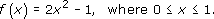This is an example of a real function, because it associates with a given real number
Author(s): The Open University

Our everyday experience of percentages includes percentage increases (like VAT at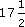%, or a service charge of 15%) and percentage decreases (such as a discount of 15%).

For example, £8 plus
Author(s): The Open University